# Novel Dominant Color Subband Image Encryption in Visual Sensor Network for Smart Military Surveillance System

Novel Dominant Color Subband Image Encryption in Visual Sensor Network for Smart Military Surveillance System
Department of ECE, Saveetha School of Engineering, Saveetha Institute of Medical and Technical Sciences, Tamil Nadu 602105, India

Saveetha Institute of Medical and Technical Sciences, Tamil Nadu 602105, India

Corresponding Author Email:
nithyar.sse@saveetha.com
Page:
951-960
|
DOI:
https://doi.org/10.18280/ts.390322
13 May 2022
|
Accepted:
12 June 2022
|
Published:
30 June 2022
| Citation

OPEN ACCESS

Abstract:

Internet of Things plays a major role in improving the levels of intelligence for several defense and military applications. There are myriads of visual sensor nodes deployed in various domain areas to acquire complete situational awareness. These sensor nodes must transmit the real-time information obtained from multiple locations to identify the threats in the danger-prone zones. The keyframes obtained from the visual sensor nodes should reach the command and control room in a confidentially manner. The lightweight scheme proposed for encryption in the visual sensor network is the novel Dominant color subband image encryption. This scheme involves encrypting only the dominant subband from an entire RGB image using the novel hybrid Chaotic method. The hybrid chaotic method combines the distinctive features of Quadratic, Ginger breadman, and Baker’s map. This hybrid scheme develops a complex, diverse chaotic structure that is unpredictable. A comparative analysis of this encryption scheme against other chaotic methods was done. It was found that the Novel Dominant color subband image encryption algorithm performs significantly better than the other existing methods to achieve real-time security in vision sensors. This method takes advantage of having lesser computation time and increased PSNR values, which indicates the higher quality of the images after decryption.

Keywords:

military surveillance, visual sensor network, image encryption, chaotic method, color subband, baker’s map, quadratic, ginger breadman

1. Introduction

Security of the visual content captured through the sensor nodes deployed in the various zones of the Visual sensor network and the unmanned vehicles is a major concern in the military surveillance IoT system. Many cryptographic algorithms were employed to achieve the confidentiality of the visual data.

The important aspect of those algorithms was that there usually occurs a tradeoff between the level of security achieved and the time of computation and resource utilization. The encryption algorithms are basically of two types.

The first type uses a similar key for performing encryption on the sender side and decryption on the receiver side, whereas the other uses different keys. In Asymmetric encryption, there occurs a public and a private key to increase the level of security. The vision sensor nodes were preprocessed for conversion from the real-time video into the number of key frames. The keyframes with meaningful information must be appropriately encrypted and then transferred to the command and control center.

The keyframes are nothing but the image, which is the 2-dimensional representation of information in the form of pixels. Since the pixels in the matrix are highly correlated, and the data capacity is very high, Data Encryption Standard-based encryption is not suitable for keyframe encryption . The Advanced Encryption Standard method showed increased confidentiality along with the reduced speed of computation by parallel processing and flexibility in selecting the different length secret keys . To optimize the time efficiency, a selective portion of the keyframe is encrypted using Discrete Cosine Transform .

## 1.png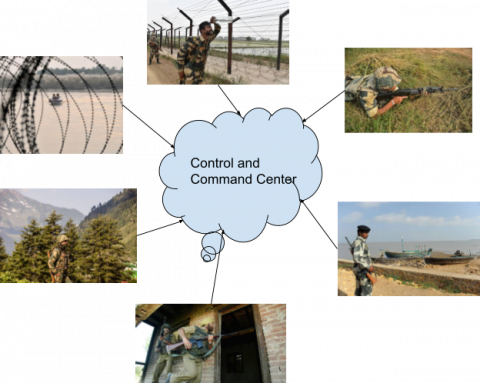Figure 1. Visual sensor network with sensors deployed in hotspots of smart military surveillance system

The deployment of sensor nodes in the military surveillance system is shown in Figure 1. The keyframes obtained from the real-time surveillance camera nodes of various areas such as landside country’s border, hillside border, watery borders like a river, lakes, etc., and housing regions near the country’s border are encrypted and transmitted to the control and command center. The encryption algorithm should satisfy the requirements such as speed of the encryption process, resistance against the static and differential attacks, and efficiency of the information delivery. The randomness of the encryption algorithm can be achieved through a Cellular automation scheme. This mechanism uses several rules for performing the permutation and diffusion techniques . The dynamic behavior can further be increased by the Chaos-based encryption mechanisms. There exist several chaotic schemes over different dimensions to minimize the correlation between the pixels in the image. Even the minute change in the initial conditions showed a major deviation in shifting the pixels and thus increased the randomness .

Several Chaotic schemes were efficient in showing higher resistance to differential attacks and having higher entropy values . But it takes more time to complete the encryption process. The limitation of the existing methods was that if the strength of the encryption increases, the speed of computation gets reduced. In this research work, the novel scheme of Dominant color subband image encryption is proposed to speed up the encryption process in the visual sensor network without sacrificing the strength of the encryption technique. Experimental results show that the system provide better performance with a lesser computation time of 16s and with a higher PSNR of 25db.

This paper discusses the previous related works done to speed up the encryption process. The three chaotic schemes, such as the Baker map, Quadratic map, and Gingerbreadman map were briefly discussed with the chaotic distribution and encryption process. Two novel schemes were proposed in section 3:

1) Novel Hybrid Scattering Chaotic method and

2) Novel Dominant color subband encryption.

The algorithm used for the encryption process is the novel hybrid Scattering Chaotic map which further increases encryption’s strength, making it to be an efficient scheme  compared with any other existing schemes.

Section 4 compares the performance of all schemes using keyspace analysis, key sensitivity analysis, analysis of correlation coefficients, effects of noise, Entropy analysis, and Speed analysis. Statistical analysis was also done using IBM SPSS statistical tool.

Section 5 concludes the results and discussions. The strength and speed of the encryption were inversely proportional to the existing algorithms consistently, making them to be less efficient. The novel dominant color subband encryption technique attains a higher key sensitivity and lesser computation time, thus changing the strength and the speed relationship. It is more important to consider the entropy levels of different algorithms for a better comparison. It also concluded that the novel dominant color subband encryption was found to be an efficient scheme compared to other methods.

In this research work, the novel scheme of dominant color subband image encryption is proposed to speed up the encryption process in the visual sensor network. The algorithm used for the encryption process is the novel hybrid scattering chaotic map which further increases the strength of the encryption, making it to be an efficient scheme  comparison with any other existing schemes.

2. Related Works

Many existing real-time image encryption algorithms involve the process of image compression, and then the encoded image is split out into significant and insignificant portions. The portions of the important bits in each pixel for encryption were selected to obtain the unrecognizable image [7, 8]. Proposed the concept of dividing the image into two frequency parts using a wavelet coding method and encrypting only the lower frequency part to reduce the encryption time. Another method of partial image encryption was done by selecting the desired coefficients using particle swarm optimization, and it was converted into a cipher image using Daubechies transform. It also consumes more time to complete the transformation process. It showed better resistance to statistical attacks, but it was achieved through several rounds of Permutation . The pixel-based partial encryption scheme is discussed in the study of Goel and Chaudhari . In this method, the RGB image was divided into several blocks and the median of the blocks of pixels was chosen as the criterion for selective encryption because the median of the pixels must contain all the important features and edges information of an image. The limitation here was the computational complexity involved in the application of masks. The thresholding mechanism to select the bitplane is discussed in the study of Li et al. , which contains more visual information than other bitplanes. The drawback of this scheme is that if more than one bitplane shows sensitive information, then it will not provide efficient encryption output.

Real-time image encryption was possible by encrypting the partial portion of the image based on the percentage of encryption requirements. The partial area of an image was selected using the Logistic Sitemap. The encryption process was dynamically varied based on the real-time needs. The percentage of encryption depends on the threshold value, considered the control parameter . Another real-time image encryption proposed by Pandurangi Ramacharya et al.  used the automatic selection of areas to be encrypted in which the partial areas were selected through the application of Fuzzy rules. This method seemed to be faster compared to the standard encryption techniques. To further reduce the computation time and speed up the encryption process, partial encryption is performed by selecting only one of the dominant color bands for encryption, leaving away the remaining bands. It is necessary to prove that the information is delivered efficiently even by sending only a single band compared to transmitting the entire RGB image.

3. Proposed Work

3.1 Hybrid scattering chaotic method

The basic idea behind chaotic encryption is to use a few dynamic structures to generate a series of numbers that can be random. Dynamic structures used for encryption cannot be recovered quickly without the particular key. Still, it can bring back to normal form by providing accurate initial conditions. The intruder cannot be able to guess the specific initial conditions to get around the original RGB image. The complexity of the chaotic techniques depends on the random shuffling of the dynamic structures and the number of dimensions in which chaotic nature is employed. Such kind of dynamic structures was used for encrypting the image. There are several 2D chaotic maps existing to perform image encryption. The randomness and strength of the key generation vary based on the design parameters. The novel hybrid scattering chaotic method combines the characteristics of Baker, Quadratic and Gingerbread Man chaotic algorithms.

3.1.1 Baker’s map

## 2.png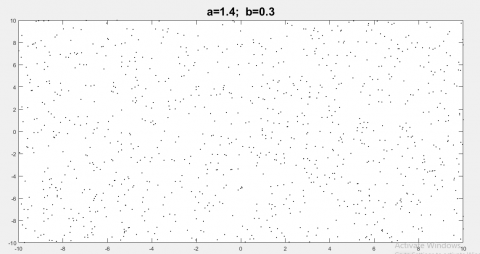Figure 2. Baker’s map chaotic distribution obtained for the parameters a=1.4 and b=0.3

## 3.png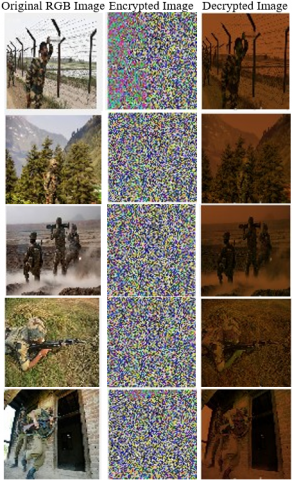Figure 3. Baker chaotic method of image encryption for real-time images from Visual sensor network

The Baker map unpredictably diffuses the image, going horizontally to the end of the block and then to the row above the current row. The baker function is below, where a and b denote the system parameters considered a=1.4 and b=0.3, and xn and yn denote state variables . Since the system is chaotic, two chaotic sequences xn and yn can be generated. The baker function is used to generate two random arrays of key values. One of the keys is utilized for altering the image pixel positions, and another key is used to change the value of the pixels themselves. Now, these key values are used to encrypt the image.

$x_{(n+1)}=1-a x^{2}+y$    (1)

$y_{(n+1)}=b y_{n}$     (2)

It is seen that the key generation in the x-direction depends on the previously obtained values of x and y. But the key generation in the y-direction depends only on the previous values of y and not on x. The control parameters are initialized by considering that the chosen values must be at a good point that does not terminate the iteration to the fixed point as 0. By random checking, it was found that the values of a=1.4 and b=0.3 provided better results without removing the iterations. The initial values of x, b, and y were found to be any value between 0 and 1. The Baker’s map chaotic distribution obtained is shown in Figure 2. The real-time samples are encrypted using Baker’s encryption algorithm, and the results are displayed in Figure 3.

The Quadratic map can be represented by the following equation:

$x_{n+1}=r-\left(x_{n}\right)^{2}$     (3)

where, r is the parameter that determines the randomness and n is the parameter that denotes the iterations to be performed.

## 4.png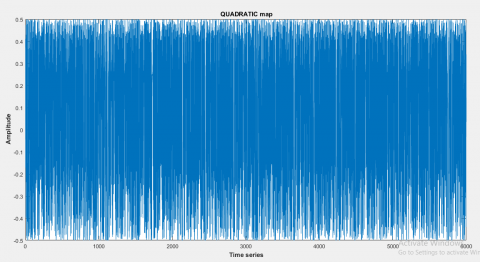Figure 4. Quadratic chaotic distribution in time domain

As the Eq. (3) gets modified to a higher level, the chaotic map's range increases, resulting in a larger key space. The quadratic map in the time domain is represented in Figure 4. This provides higher resistance to several statistical attacks . This equation is modified to matrix form for the shuffling and substitution applications as

$X(i, j)=X^{2}-Y^{2}+a X+b Y$

$Y(i, j)=2 X Y+c X+d Y$

where, a, b, c and d are the control parameters which are responsible for randomness whose values are in the range of 0 to 2. The values of X and Y should be in the range of 0 to 1. The out put of the encrypted real - time samples from the visual sensor nodes using quadratic encryption scheme are shown in Figure 5.

## 5.png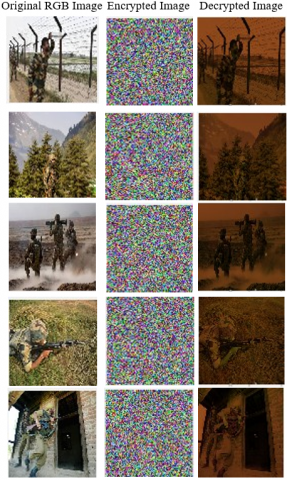Figure 5. Quadratic chaotic encryption for the real-time sample images from Visual Sensor Network

## 6.jpg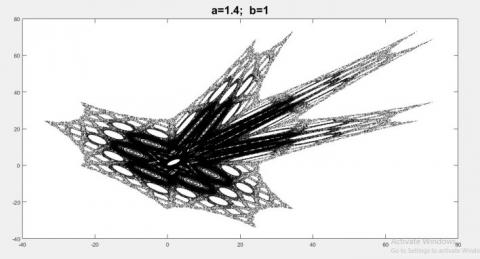Figure 6. Gingerbread man chaotic distribution in transform domain

The Gingerbread man function is given below as Eq. (4) and Eq. (5), where xn and yn denote state variables. Since the system is chaotic, two chaotic sequences, xn and yn can be generated. The gingerbread man function generates two random arrays of key values. These key values are used to encrypt the image.

$X_{(n+1)}=1-y_{n}+\left|X_{n}\right|$    (4)

$y_{(n+1)}=X_{n}$     (5)

This mapping structure contains six hexagonal sections to represent the dispersed chaotic behavior . It also shows linearity and stable performance in some regions and randomness in others. So, it is a piece-wise linear 2-dimensional chaotic map. It is represented in Figure 6. The gingerbread man encrypted sample images are displayed in Figure 7.

## 7.png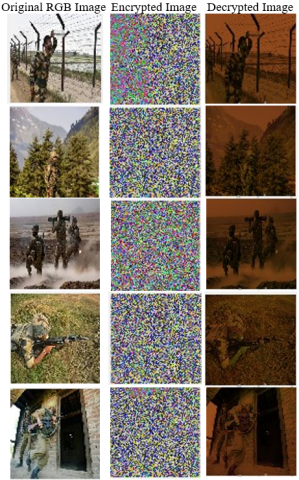Figure 7. Gingerbread man chaotic image encryption in visual sensor network

3.1.4 Combined hybrid scattered chaotic method

The Hybrid Scattered Chaotic Method of image encryption involves the distinctive features of Baker, Quadratic and Gingerbread man maps to generate of key streams. The initial parameters chosen to be a=0.9, b=0.6, c=2 and d=0.50. The key streams for the sub-situation matrix are created using Baker’s map kneading operation. The values of the pixels get changed according to the rule of Baker’s map along the x-direction. Then the values along the x-direction are directly mapped to the y-direction using the Quadratic mapping rules. Finally, the key stream for the diffusion process is generated using the Gingerbread Man Chaotic map algorithm. The values generated for the diffusion process seem to be random in some parts of the matrix and stable in other parts of the matrix. This type of mixing increases the randomness behavior without satisfying the time taken for encryption. The combined map obtained is shown in Figure 8.

## 8.jpg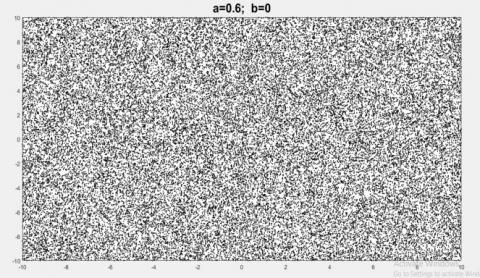Figure 8. Hybrid chaotic map distribution for the parameters a=0.6 and b=0

Steps involved in Encryption Process:

The key frames obtained from the sensor nodes can be converted into their RGB components.

1) The first step is resizing of the original RGB image or keyframes into three color matrices, which are considered to be the vectors of integers ranging between 0 and 512. The entire plain image can be modified as P={RMatrix, GMatrix, BMatrix}.

2) Each matrix is processed separately for encryption and then finally combined to obtain the Cipher image.

3) The key stream Sequence obtained from the hybrid mixing of Baker and the Quadratic map is used as the shuffling matrix.

4) The position of the pixels in the color matrices gets shuffled according to the shuffling matrices.

5) After Shuffling, the key stream sequence generated using Gingerbread man Map is used toper form the diffusion.

6) Bitwise XOR operation is performed along the pixels of the color matrices. The length of the key is 64-bits.

7) To equalize the size of the color matrices with the key stream matrix, padding is to be done.

8) Here, the color matrices are padded with 1, and then it is bitwise XOR with the key stream matrix generated using the Gingerbread man chaotic map.

9) Finally, their resultant matrices are converted into unsigned integer matrices.

All the three integer matrices are combined to obtain a Cipher image. The output of the novel hybrid chaotic method is shown in Figure 9.

3.2 Novel dominant color subband image encryption

The main objective of this research is to lower the encryption time without the loss of information along with the confidentiality of the Visual data. The visual information captured through the camera nodes was usually converted into RGB color frames, and each of the keyframes was encrypted for security purposes. The entire RGB color frame requires more resources for computation of the encryption process, and also it needs more time. But the confidential data has to be reached the control room within a short period. Instead of performing the encryption on the overall RGB color frame, Partial image encryption needs to be done. At the same time, the process has to completely retrieve all the important information present in the color frame to the control room.

To achieve this, the RGB color frames are split out into three numbers of color subbands, and the algorithm is used to determine the dominant color subband of an image. Then the Dominant color subband alone is encrypted and transmitted to the control room.

## 9.pngFigure 9. Novel hybrid scattered chaotic image encryption in visual sensor network

## 10.pngFigure 10. Flowchart of novel dominant color subband image encryption

Steps involved in the Encryption process:

Step 1: The Visual data is converted into standard RGB Color Keyframes of 512 x 512 as the size of the image.

Step 2: The RGB color key frames are pre-processed to determine the mean of the individual color subband.

Step 3: The subband with the dominant mean value will be considered the dominant color subband, and it is further used for the encryption process.

Step 4: The encryption is performed using the novel hybrid scattering chaotic method on the dominant color subband. The Flowchart for the overall Encryption mechanism is shown in Figure 10. The output of the novel dominant color subband encryption obtained for the real-time sample images is shown in Figure 11.

## 11.pngFigure 11. Novel dominant color sub band image encryption in visual sensor network

4. Performance and Security Analysis

Several statistical and differential tests have been performed to evaluate the Novel hybrid scattering chaotic encryption and the Novel dominant color subband image encryption.

4.1 Key space analysis

The key space plays an important role in determining the security level of the image encryption techniques. If the particular encryption scheme shows more chaotic and random behavior, it provides better security. This can be achieved by increasing the key space. From the previous studies, it has been found that the algorithm is found to be more secure if it has a key space greater than 2100 . The key space can be analyzed based on the algorithm's sensitivity to the initial conditions. The initial conditions assumed for a total often variables with the precision of 10-16. Thus, the total key space is (1016)10=10160, which is highly higher to resist Brute force attacks.

4.2 Key sensitivity analysis

The security of the cryptographic algorithm should be more sensitive, even to a slight change occurring in the secret keys. This small change in the key used for decryption results in a different image almost similar to the cipher image but not to the original image. The following parameters can analyze the key sensitivity: Number of Pixels of Change Rate (NPCR) and Unified averaged Changed Intensity (UACI). The sensitivity of the encryption can be determined by changing the pixels of the image or by changing the values of the key . So, the image encryption should be more sensitive to both the image and the key. The key sensitivity can be obtained by encrypting the image two times. First, it is encrypted by the actual key generated by the chaotic algorithm, which is considered to be C1. It is again encrypted by the modified values of the key, which is regarded as C2 . The image sensitivity can be obtained by encrypting the original image for the first time. Then the least significant pixels of the images are modified and encrypted for the second time. The values of NPCR and UACI can be evaluated using Eqns. (6) and (7).

$M^{*} N$

$N P C R=\left[\sum D(x) / M^{*} N\right] * 100 \%$

$x=1$

$\left\{\begin{array}{l}D(x)=\{1 C 1(x) \neq C 2(x) \\ 0 \quad C 1(x)=C 2(x)\end{array}\right.$      (6)

$U A C I=1 / M^{*} N$

$M^{*} N$

$\sum \mid C 1(x)-C 2(x) / 255 * 100$

$x=1$       (7)

Table 1 compare of NPCR and UACI values of Baker, Quadratic, Gingerbread man, Hybrid, and dominant color subband schemes.

4.3 Correlation coefficients

The Correlation between the two adjacent pixels can be used to identify the strength of the encryption technique. If the encryption is considered good, it must show the non-linear relationship between the adjacent pixels with values of correlation coefficients closer to zero. The correlation coefficients can be determined by fixing the length of the sequence N in all directions. The original image before encryption has correlation coefficient values closer to 1 in all directions.

It is found that the hybrid encryption scheme, as well as the novel dominant color subband image encryption algorithm obtained the correlation coefficient values closer to zero in all directions, which further denote the increased strength of the image encryption scheme. The proposed scheme is secure against statistical attacks. The cross-correlation between the adjacent pixels can be obtained by finding the covariance and variance of the adjacent pixels. It is given by Eq. (8). Figure 12 shows the cross correlation of the five different chaotic schemes in horizontal, vertical and diagonal directions.

$C(x, y)=\operatorname{cov}(x, y) /(\sqrt{\operatorname{var}(x) \sqrt{\operatorname{var}(y))}}$

where, x and y represent the gray values of two adjacent pixels.

$M*N$

$\operatorname{Cov}(x,y)=1/M*N\left( \sum{\left( {{x}_{i}}-E(x) \right)}\left( {{y}_{i}}-(y) \right) \right.$

i=1

$E(x)=1/M*N_{\sum{xi}}^{M*N}i=1$

$\operatorname{var}(x)=1/M*N$$M*N$          (8)

From Table 2 for cross–correlation shows that the two novel schemes achieved the cross- correlation values nearer to zero in horizontal, vertical and diagonal directions and were the stronger encryption methods.

4.4 Effect of noise

The standard of the decrypted image at the output side can be determined by the parameter called Peak Signal to Noise Ratio (PSNR) . It is given by the Eq. (9),

$M-1,N-1$

$PSNR=10\log {{M}^{*}}N{{(255)}^{2}}/\sum{{{\left( {{f}_{1}}(i,j)-{{f}_{2}}(i,j) \right)}^{2}}}$

$i=0,j=0$        (9)

Table 1. NPCR and UACI

 SampleImage512x512 BAKER’S QUADRATIC GINGERBREADMAN HYBRID DOMINANTSUBBAND NPCR % UACI % NPCR % UACI % NPCR % UACI % NPCR % UACI % NPCR % UACI % 1 99.60 29.94 99.62 29.99 99.60 29.94 99.60 29.89 99.60 35.47 2 99.59 31.88 99.61 31.89 99.59 31.88 99.60 31.97 99.62 37.84 3 99.60 32.19 99.61 32.27 99.60 32.19 99.59 32.25 99.61 37.84 4 99.62 31.83 99.61 31.77 99.62 31.83 99.59 31.94 99.60 37.98 5 99.61 35.17 99.62 35.19 99.61 35.17 99.60 35.32 99.62 40.71

Table 2. Cross correlation

 SampleImage512x512 BAKER’S QUADRATIC GINGERBREADMAN HYBRID DOMINANT SUBBAND R G B R G B R G B R G B R 1 4.35x10-4 -0.0035 6.45x10-5 -0.0029 -0.0032 0.0030 4.36x10-4 -0.0035 6.45x10-5 0.0050 4.06x10-4 -0.0067 3.63x10-4 2 -0.0027 -0.0024 -0.0026 3.36x10-4 -0.0012 0.0049 -0.0027 -0.0024 -0.0026 1.21x10-4 -0.0010 -0.0058 -0.0010 3 -0.0035 -0.0015 -0.0037 -0.0055 -1.81x10-5 0.0034 -0.0035 -0.0015 -0.0037 0.0037 0.0035 0.0016 0.0035 4 -0.0021 -0.0028 -0.0024 1.36x10-4 -4.49x10-4 0.0012 -0.0021 -0.0028 -0.0024 0.0044 8.29x10-4 -0.0016 8.194x10-4 5 0.0029 0.0016 -8.98x10-4 0.0011 0.0014 0.0029 0.0029 0.0016 -8.98x10-4 -7.04x10-4 -0.0011 0.0041 -0.0011

## 121.png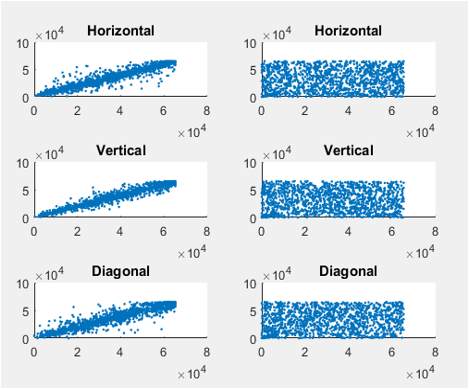(a) Baker’s method

## 122.png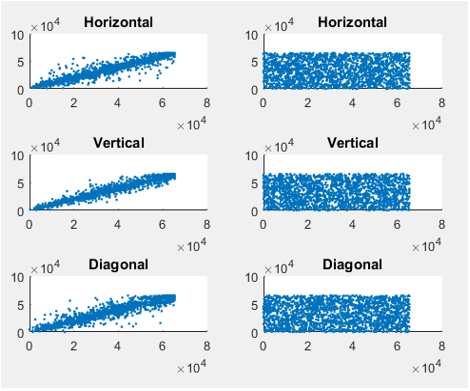## 123.png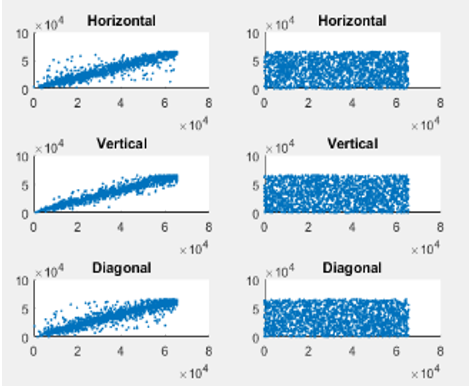## 124.png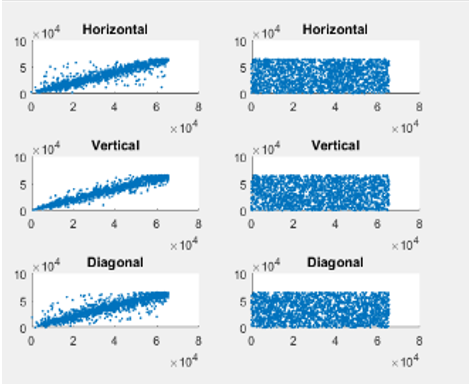(d) Novel hybrid method

## 125.png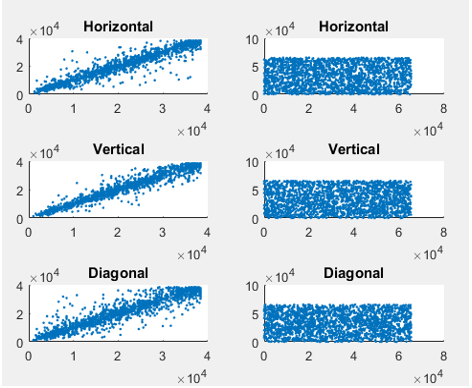(e) Novel dominant color subband method

Figure 12. Cross correlation relation of the pixels in the respective directions for the five different chaotic schemes

where, f1 (i, j) represents the value of the pixel obtained from the original image and f2 (i, j) represents the value of the pixel obtained from the decrypted image. The value of the PSNR is found to be higher for the efficient encryption scheme . From Table 3 of PSNR, it is found that the PSNR values of Novel dominant color subband encryption are higher than the other chaotic methods such as Baker, Quadratic, and Gingerbread man encryption schemes. These characteristics strongly proved that the quality of the decrypted output is better than the other schemes.

4.5 Information entropy analysis

Entropy is the randomness of the information obtained from the average of pixels available in an image . The pixels in an image whose values range from 0 to 255 must have the theoretically calculated entropy value of 8 . It is given by the Eq. (10),

$H(x)=\sum\limits_{i=1}^{n}{p}\left( {{x}_{i}} \right){{\log }_{2}}p\left( {{x}_{i}} \right)$     (10)

where, p(xi) denotes the probability of xi. Table 4 for entropy clearly proves that all the 5 encryption schemes achieved the value of 8.

4.6 Speed analysis

Other factors also seem to be more important when doing image encryption in real-time . Speed analysis is essential for real-time multimedia image encryption . The execution time required to process an encryption can be evaluated for all algorithms only when they are operated under the same environment. All trial results were performed on CPU 7th gen, i5, 8 GB RAM, 500 GB HDD, installed with Matlab variant R2018b. From Table 5 of computation time, it is found that the Novel Dominant color subband encryption scheme consumes very less time to perform the real-time image encryption process compared to all the other schemes.

4.7 Statistical analysis using SPSS

The computation time of the different encryption algorithms can be analyzed using the IBMSPSSV26.0 Statistical analysis software . The test performed was the one-way Anova T- Test. The dependent variable is the computation time, and the independent variable is the size of the image. Table 6 and Table 7 show that the novel color subband image encryption provided better results compared to the chaotic methods, with a significance of 0.0001(<0.05) concerning time. Figure 13 shows the simple bar mean of five different chaotic methods.

Table 3. PSNR

 Sample Image 512 x 512 Baker’s Quadratic Ginger breadman Hybrid Dominant subband R G B R G B R G B R G B R 1 14.78 14.22 13.19 15.49 14.98 13.98 14.79 14.21 13.19 13.56 12.99 12.04 13.93 2 12.53 11.81 7.97 10.16 10.68 11.59 12.53 11.80 9.76 13.26 12.49 10.31 23.48 3 12.26 11.44 10.87 12.06 11.25 10.69 12.27 11.44 10.87 13.56 12.64 12.01 23.66 4 12.31 11.57 9.027 10.21 9.56 7.44 12.32 11.57 9.03 13.7 12.9 10.06 25.13 5 10.19 10.20 9.60 14,41 14.51 13.93 10.19 10.20 9.60 11.43 11.46 10.82 20.32

Table 4. Entropy

 Sample Image 512 x 512 Entropy of Original Image Entropy of BAKER’S method Entropy of Quadratic method Entropy of Ginger bread man method Entropy of Hybrid method Entropy of Dominant color subband 1 7.6565 7.9997 7.9997 7.9997 7.9997 7.9993 2 7.7247 7.9997 7.9997 7.9997 7.9997 7.9991 3 7.7050 7.9997 7.9997 7.9997 7.9997 7.9993 4 7.6015 7.9997 7.9997 7.9997 7.9997 7.9991 5 7.4677 7.9997 7.9997 7.9997 7.9997 7.9991

Table 5. Computation time

 SampleImage512x512 BAKER’S method Quadratic method Gingerbread man method Hybrid method Dominant color subband. 1 62.91 s 59.95s 58.74s 56.29s 15.98 s 2 59.03s 59.34 s 60.17 s 58.43s 16.15 s 3 56.75s 64.31s 55.51s 58.72s 15.89s 4 58.52s 62.00s 56.51 57.18s 16.57s 5 61.27s 62.28 s 64.01s 53.92s 16.62s

Table 6. One-way ANOVA T-test

 Descriptives N Mean Std. deviation Std. Error Lower Bound Upper Bound Minimum Maximum BAKER 5 59.71 2.446 1.094 56.67 62.5 57 63 QUADRATIC 5 61.58 1.987 .889 59.11 64.04 59 64 GINGERBREADMAN 5 58.99 3.351 1.499 54.83 63.15 56 64 HYBRID 5 56.91 1.935 .866 54.50 59.31 54 59 DOMINANT SUBBAND 5 16.24 .336 .150 15.82 16.66 16 17 TOTAL 25 50.68 17.760 3.552 43.35 58.02 16 64

Table 7. ANOVA

 ANOVA Sum of Squares df Mean Square F Sig. Between Groups 7470.071 4 1867.518 373.188 0.000 Within Groups 100.085 20 5.004 Total 7570.156 24

## 13.pngFigure 13. Simple bar mean of time by methods

5. Conclusions

The real-time military surveillance images obtained from Visual Sensor Network are encrypted securely and high speed using novel dominant subband image encryption The quality of the decrypted image was also better with higher values of PSNR. The algorithm used here for novel dominant subband image encryption is the hybrid Chaotic scheme which provides better performance against statistical attacks than other chaotic encryption schemes. Experimental results and the theoretical analysis also proved that the above scheme is significantly better in performing image encryption with a lesser computation time of 16s and with a higher PSNR of 25Db in the Visual Sensor Network.

References

 Subramanyan, B., Chhabria, V.M., Babu, T.S. (2011). Image encryption based on AES key expansion. In 2011 Second International Conference on Emerging Applications of Information Technology, Kolkata, pp. 217-220. https://doi.org/10.1109/EAIT.2011.60

 Radhadevi, P., Kalpana, P. (2012). Secure image encryption using AES. International Journal of Research in Engineering and Technology, 1(2): 115-117.

 Akram, B., Oussama, B., Houcemeddine, H., Safya, B. (2014). Selective image encryption using DCT with AES cipher. In Proceedings of the NETCOM, pp. 69-74. https://doi.org/10.5121/csit.2014.41306

 Chen, T., Zhang, M., Wu, J., Yuen, C., Tong, Y. (2016). Image encryption and compression based on kronecker compressed sensing and elementary cellular automata scrambling. Optics & Laser Technology, 84: 118-133. https://doi.org/10.1016/j.optlastec.2016.05.012

 Fan, H., Zhang, C., Lu, H., Li, M., Liu, Y. (2021). Cryptanalysis of a new chaotic image encryption technique based on multiple discrete dynamical maps. Entropy, 23(12): 1581. https://doi.org/10.3390/e23121581

 Mokhnache, A., Ziet, L. (2020). Cryptanalysis of a pixel permutation based image encryption technique using chaotic map. Traitement du Signal, 37(1): 95-100. https://doi.org/10.18280/ts.370112

 Hazarika, N., Borah, S, Saikia, M. (2014). A wavelet based partial image encryption using chaotic logistic map. In 2014 IEEE International Conference on Advanced Communications, Control and Computing Technologies Ramanathapuram, India, pp. 1471-1475. https://doi.org/10.1109/ICACCCT.2014.7019347

 Xiang, T., Wong, K.W., Liao, X. (2007). Selective image encryption using a spatiotemporal chaotic system. Chaos: An Interdisciplinary Journal of Nonlinear Science, 17(2): 023115. https://doi.org/10.1063/1.2728112

 Kuppusamy, K., Thamodaran, K. (2012). Optimized partial image encryption scheme using PSO. In International Conference on Pattern Recognition, Informatics and Medical Engineering (PRIME-2012), Salem, India, pp. 236-241. https://doi.org/10.1109/ICPRIME.2012.6208350

 Goel, A., Chaudhari, K. (2016). Median based pixel selection for partial image encryption. In 2016 Sixth International Conference on Image Processing Theory, Tools and Applications (IPTA), Oulu, Finland, pp. 1-5. IEEE. https://doi.org10.1109/IPTA.2016.7820931

 Li, J., Zhang, Z., Li, S., Benton, R., Huang, Y., Kasukurthi, M.V, Huang, J. (2020). A partial encryption algorithm for medical images based on quick response code and reversible data hiding technology. BMC Medical Informatics and Decision Making, 20(14): 1-16. https://doi.org/10.1186/s12911-020-01328-2.

 Parameshachari, B.D. (2021). Logistic sine map (LSM) based partial image encryption. In 2021 National Computing Colleges Conference (NCCC), Taif, Saudi Arabia, pp. 1-6. https://doi.org/10.1109/NCCC49330.2021.9428854

 Pandurangi Ramacharya, B., Patil, M.R., Keralkar, S. (2022). Fast partial image encryption with fuzzy logic and chaotic mapping. Evolutionary Intelligence. https://doi.org/10.1007/s12065-021-00693-9

 Saravanan, S., Sivabalakrishnan, M. (2020). An image encryption scheme using chaotic baker map. Journal of Computational and Theoretical Nanoscience, 17(5): 2130-2135. https://doi.org/10.1166/jctn.2020.8859

 Herbadji, D., Belmeguenai, A., Derouiche, N., Liu, H. (2020). Colour image encryption scheme based on enhanced quadratic chaotic map. IET Image Process., 14(1): 40-52. https://doi.org/10.1049/iet-ipr.2019.0123

 Khan, M., Asghar, Z. (2018). A novel construction of substitution box for image encryption applications with Gingerbreadman chaotic map and S8 permutation. Neural Computing and Applications, 29(4): 993-999. https://doi.org/10.1007/s00521-016-2511-5

 Jan, A., Parah, S.A., Malik, B.A. (2022). IEFHAC: Image encryption framework based on Hessenberg transform and chaotic theory for smart health. Multimedia Tools and Applications, 81(13): 18829-18853. https://doi.org/10.1007/s11042-022-12653-1

 Ali, T.S., Ali, R. (2022). A novel color image encryption scheme based on a new dynamic compound chaotic map and S-box. Multimedia Tools and Applications, 81: 20585-20609. https://doi.org/10.1007/s11042-022-12268-6

 Masood, F., Ahmad, J., Shah, S.A., Jamal, S.S., Hussain, I. (2020). A novel hybrid secure image encryption based on Julia set of fractals and 3D Lorenz chaotic map. Entropy, 22(3): 274. https://doi.org/10.3390/e22030274

 Sarosh, P., Parah, S.A., Bhat, G.M. (2022). An efficient image encryption scheme for healthcare applications. Multimedia Tools and Applications, 81: 7253-7270. https://doi.org/10.1007/s11042-021-11812-0

 Zhu, C., Wang, G., Sun, K. (2018). Improved cryptanalysis and enhancements of an image encryption scheme using combined 1D chaotic maps. Entropy, 20(11): 843. https://doi.org/10.3390/e20110843

 Askar, S.S., Karawia, A.A., Al-Khedhairi, A., Al-Ammar, F.S. (2019). An algorithm of image encryption using logistic and two-dimensional chaotic economic maps. Entropy, 21(1): 44. https://doi.org/10.3390/e21010044

  Hosny, K.M. (2020). Multimedia Security Using Chaotic Maps: Principles and Methodologies. New York: Springer. https://doi.org/10.1007/978-3-030-38700-6

 Raman, A. (2016). Parallel processing of chaos-based image encryption algorithms. University of California, Irvine.

 McCormick, K., Salcedo, J. (2017). SPSS Statistics for Data Analysis and Visualization. John Wiley & Sons.